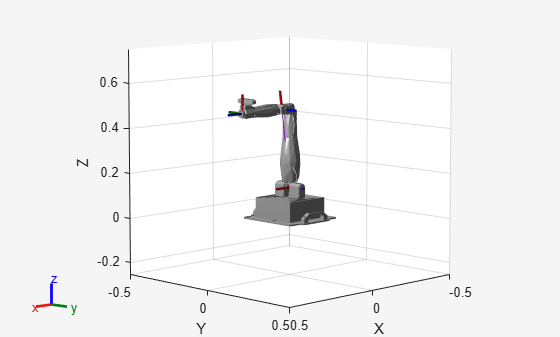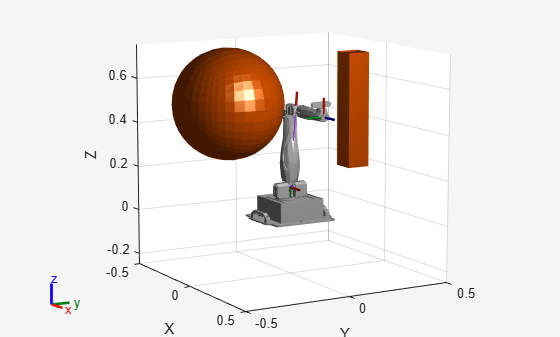# manipulatorCollisionBodyValidator

Validate states for collision bodies of rigid body tree

Since R2021b

## Description

The `manipulatorCollisionBodyValidator` object performs state and motion validity checks for a rigid body tree robot model. To check if the collision bodies collide either with other bodies (self-collisions) or the environment, use the `isStateValid` object function. To check if a motion between two states is valid, use the `isMotionValid` object function.

## Creation

### Syntax

``manipSV = manipulatorCollisionBodyValidator``
``manipSV = manipulatorCollisionBodyValidator(ss)``
``manipSV = manipulatorCollisionBodyValidator(ss,Name=Value)``

### Description

````manipSV = manipulatorCollisionBodyValidator` creates a state validator with default values for a `manipulatorStateSpace` object.```

example

````manipSV = manipulatorCollisionBodyValidator(ss)` creates a state validator for a `manipulatorStateSpace` object that represents a robot model state space and contains collision bodies for rigid body elements. Specify `ss` as a `manipulatorStateSpace` object.```
````manipSV = manipulatorCollisionBodyValidator(ss,Name=Value)` specifies Properties as name-value arguments```

## Properties

expand all

Distance resolution for motion validation, specified as a positive scalar. The validation distance determines the number of interpolated states between states specified to the `isMotionValid` object function. The object function validates each interpolated state by checking for collisions with the robot and the environment.

Data Types: `double`

Ignore self collisions toggle, specified as a logical. If this property is set to `true`, the `isMotionValid` object function skips checking between bodies for collisions and only compares the bodies to the environment. Not checking for self-collisions can improve the speed of the planning phase, but your state space should contain joint limits that ensure self-collisions are not possible.

Data Types: `logical`

Collision objects in the robot environment, specified as a cell array of collision objects of these types:

```box = collisionBox(0.1,0.1,0.5); % XYZ Lengths box.Pose = trvec2tform([0.2 0.2 0.5]); % XYZ Position sphere = collisionSphere(0.25); % Radius sphere.Pose = trvec2tform([-0.2 -0.2 0.5]); % XYZ Position env = {box sphere}; manipSS = manipulatorCollisionBodyValidator(ss,Environment=env);```

Data Types: `logical`

Manipulator state space, specified as a `manipulatorStateSpace` object, which is a subclass of `nav.StateSpace` (Navigation Toolbox).

Body pairs skipped for checking self-collisions, specified as either `"parent"` or `"adjacent"`:

• `"parent"` — Skip collision checking between child and parent bodies.

• `"adjacent"` — Skip collision checking between bodies on adjacent indices.

Data Types: `char` | `string`

## Object Functions

 `isStateValid` Check if state is valid `isMotionValid` Check if path between states is valid

## Examples

collapse all

Generate states to form a path, validate motion between states, and check for self-collisions and environmental collisions with objects in your world. The `manipulatorStateSpace` object represents the joint configuration space of your rigid body tree robot model, and can sample states, calculate distances, and enforce state bounds. The `manipulatorCollisionBodyValidator` object validates the state and motion based on the collision bodies in your robot model and any obstacles in your environment.

Use the `loadrobot` function to access predefined robot models. This example uses the Quanser QArm™ robot and joint configurations are specified as row vectors.

```robot = loadrobot("quanserQArm",DataFormat="row"); figure(Visible="on") show(robot); xlim([-0.5 0.5]) ylim([-0.5 0.5]) zlim([-0.25 0.75]) hold on```Configure State Space and State Validation

Create the state space and state validator from the robot model.

```ss = manipulatorStateSpace(robot); sv = manipulatorCollisionBodyValidator(ss,SkippedSelfCollisions="parent");```

Set the validation distance to `0.05`, which is based on the distance normal between two states. You can configure the validator to ignore self collisions to improve the speed of validation, but must consider whether your robot model has the proper joint limit settings set to ensure it does not collide with itself.

```sv.ValidationDistance = 0.05; sv.IgnoreSelfCollision = true;```

Place collision objects in the robot environment. Set the `Environment` property of the collision validator object using a cell array of objects.

```box = collisionBox(0.1,0.1,0.5); % XYZ Lengths box.Pose = trvec2tform([0.2 0.2 0.5]); % XYZ Position sphere = collisionSphere(0.25); % Radius sphere.Pose = trvec2tform([-0.2 -0.2 0.5]); % XYZ Position env = {box sphere}; sv.Environment = env;```

Visualize the environment.

```for i = 1:length(env) show(env{i}) end view(60,10)```Plan Path

Start with the home configuration as the first point on the path.

```rng(0); % Repeatable results start = homeConfiguration(robot); path = start; idx = 1;```

Plan a path using these steps, in a loop:

• Sample a nearby goal configuration, using the Gaussian distribution, by specifying the standard deviation for each joint angle.

• Check if the sampled goal state is valid.

• If the sampled goal state is valid, check if the motion between states is valid and, if so, add it to the path.

```for i = 2:25 goal = sampleGaussian(ss,start,0.25*ones(4,1)); validState = isStateValid(sv,goal); if validState % If state is valid, check motion between states. [validMotion,~] = isMotionValid(sv,path(idx,:),goal); if validMotion % If motion is valid, add to path. path = [path; goal]; idx = idx + 1; end end end```

Visualize Path

After generating the path of valid motions, visualize the robot motion. Because you sampled random states near the home configuration, you should see the arm move around that initial configuration.

To visualize the path of the end effector in 3-D, get the transformation, relative to the base world frame at each point. Store the points as an xyz translation vector. Plot the path of the end effector.

```eePose = nan(3,size(path,1)); for i = 1:size(path,1) show(robot,path(i,:),PreservePlot=false); eePos(i,:) = tform2trvec(getTransform(robot,path(i,:),"END-EFFECTOR")); % XYZ translation vector plot3(eePos(:,1),eePos(:,2),eePos(:,3),"-b",LineWidth=2) drawnow end```## Version History

Introduced in R2021b

expand all